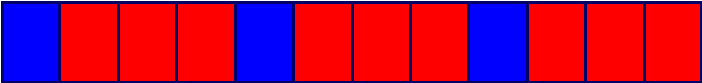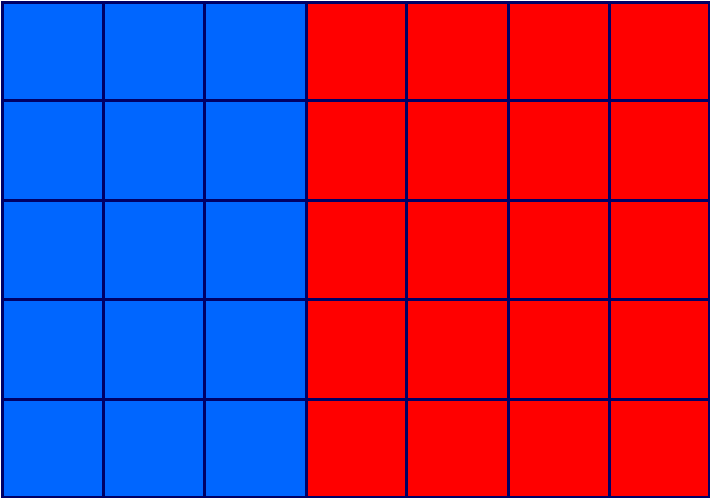# Ratio and Proportion

### Ratio's are used to compare quantities or numbers. We use the symbol ':' to separate the numbers that are being compared.

Look at this example:There are 12 squares, three are blue and nine are red. For every blue square there are three red squares. the ratio is 1 blue to 3 red, 1:3

We can also describe this pattern as a proportion. In every 4 squares, there are 3 red squares and 1 blue square. So the proportion of red squares is 3 in every 4 and the proportion of blue squares is 1 in every 4.

When you look at a number of items, a ratio compares the items to each other, 1 to 3, 1:3 whereas each item is a proportion of the total, 1 in every 4, or 3 in every 4.

### Think about these questions:  There are 35 children in a class and 15 are boys.

It may help to visualise this with a diagram, blue for boys, red for girls.## What is the ratio of boys to girls?

If there are 35 children in the class and 15 are boys, then 35 - 15 = 20 must be girls.

So the ratio of boys to girls is 15:20.

However, we can simplify a ratio in much the same way that we simplify a fraction, by dividing both sides of the ':' by the Highest Common Factor, HCF.
The HCF of 15 and 20 is 5 so divide both by 5 to give 3:4.

## What is the proportion of boys?

There are 15 boys out of a total of 35 children. So the proportion is 15 in every 35.

Again, we can simplify a proportion in much the same way that we simplify a fraction,
by the Highest Common Factor, HCF.

The HCF of 15 and 35 is 5 so divide both by 5 to give 3 and 7.

## What is the proportion of girls?

There are 20 boys out of a total of 35 children. So the proportion is 20 in every 35.

Again, we can simplify a proportion in much the same way that we simplify a fraction,
by the Highest Common Factor, HCF.

The HCF of 20 and 35 is 5 so divide both by 5 to give 4 and 7.

### We can say that the proportion of girls is 4 in every 7.

Proportion can be represented as a fraction, as a decimal or as a percentage.

The proportion of boys is 3 in every 7.

As a fraction this would be, 3 out of 7 or 3/7 - Three sevenths of the class are boys.

As a decimal, divide the numerator by the denominator, 3 ÷ 7 = 0.43 of the class are boys.

As a percentage, 3 ÷ 7 = 0.43, 0.43 x 100 = 43% - 43% of the class are boys.

Try these worksheets from Mathsblog